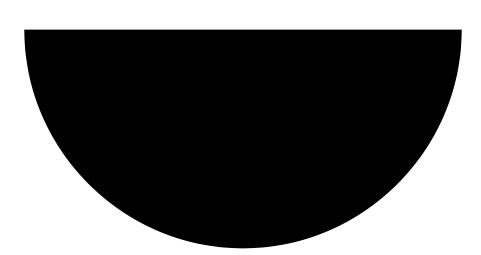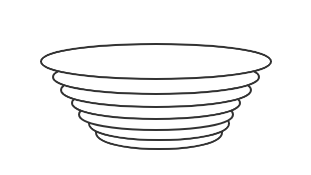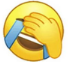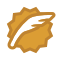# 数学思维和程序员思维//半径
var r = 5.0
//分成100份计算
var num = 100
//每份的高度, 体积
var dH = r / num, dV = 0
//圆周率
var pi = 3.14

//总功w
var w = 0
//体积
var v = 0
//计算总功和总体积
for(var i = 1; i <= num; i++) {
//距离顶部的高度
h = i * r / num
//这一段的体积
dV = (pi * (r * r - h * h) * dH)
//当前抽取的总体积
v += dV
//当前做的总功(忽略重量等因素）
w += dV * h
}

//下面和上面算法一样，但是当达到上面 w/2 的功时停止并且计算体积的比值
var w2 = 0
var v2 = 0
var percent = 0;
for(var i = 1; i <= num; i++) {
h = i * r / num
dV = (pi * (r * r - h * h) * dH)
v2 += dV
w2 += dV * h
//当达到一半功时，此时的体积占比
if (w2 > (w / 2)) {
percent = v2 / v
break
}
}
console.log('result: ', percent)isea533CSDN认证博客专家 运维开发 系统架构
《MyBatis从入门到精通》作者，MyBatis分页插件PageHelper作者，通用Mapper作者，个人网站:https://mybatis.io
06-11256409-10333
10-2061
04-067401
04-256007
08-142392
07-08752